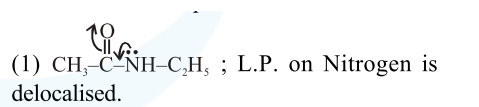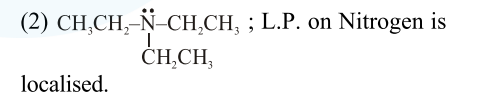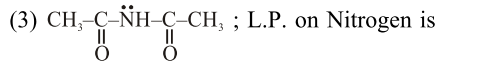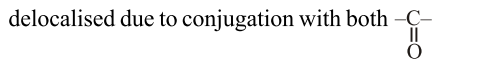# Which of the following is least basic ?Question:

Which of the following is least basic ?

1. $\left(\mathrm{CH}_{3} \mathrm{CO}\right) \ddot{\mathrm{N}} \mathrm{HC}_{2} \mathrm{H}_{5}$

2. $\left(\mathrm{C}_{2} \mathrm{H}_{5}\right)_{3} \ddot{\mathrm{N}}$

3. $\left(\mathrm{CH}_{3} \mathrm{CO}\right)_{2} \ddot{\mathrm{N}} \mathrm{H}$

4. $\left(\mathrm{C}_{2} \mathrm{H}_{5}\right)_{2} \ddot{\mathrm{N}} \mathrm{H}$

Correct Option: , 3

Solution:

For the given compounds :(Hence least basic)

(4) $\mathrm{CH}_{3}-\mathrm{CH}_{2}-\ddot{\mathrm{NH}}-\mathrm{CH}_{2}-\mathrm{CH}_{3} ;$ L.P. on Nitrogen is localised.